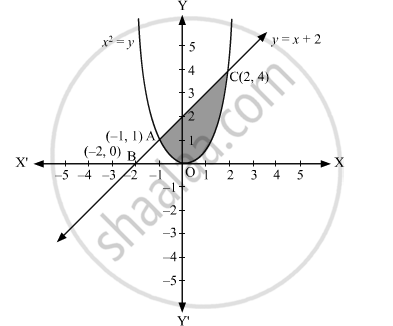# Find the Area of the Region Enclosed by the Parabola X2 = Y, the Line Y = X + 2 and X-axis - Mathematics

Find the area of the region enclosed by the parabola x2 = y, the line y = x + 2 and x-axis

#### Solution

The area of the region enclosed by the parabola, x2 = y, the line, y = x + 2, and x-axis is represented by the shaded region OACO asThe point of intersection of the parabola, x2 = y, and the line, y = x + 2, is A (–1, 1) and C(2, 4).

Area of OACO = ∫-12x + 2 dx  -  ∫-12 x2 dx⇒Area of OACO = x22 + 2x-12 - 13x3-12⇒Area of OACO = 222+22 - -122+2-1 - 1323 - -13⇒Area of OACO = 2 + 4 - 12-2 - 138 + 1⇒Area of OACO = 6 + 32 - 3⇒Area of OACO = 3 + 32 = 92 square units

Concept: Area Under Simple Curves
Is there an error in this question or solution?

#### APPEARS IN

NCERT Class 12 Maths
Chapter 8 Application of Integrals
Q 10 | Page 375